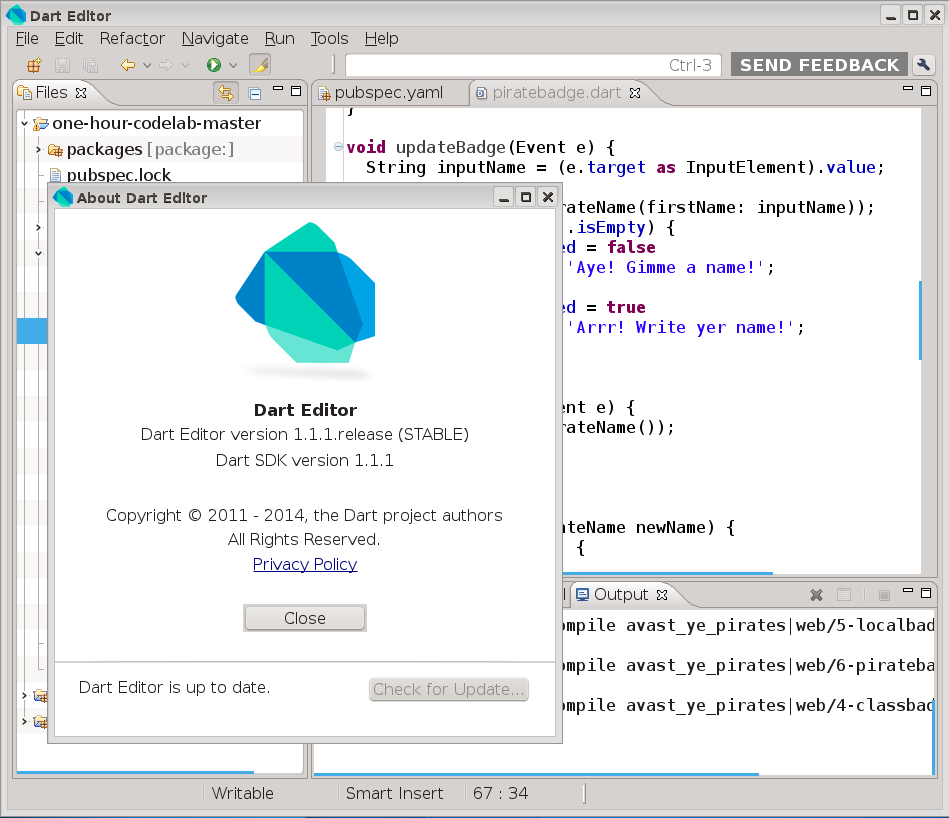# Dart 1.1.1

Version of implementation Dart of programming language Dart

A version of Dart released on January 16, 2014Dart Editor 1.1.1

## Examples:

### Hello, World! - Dart (485):

The “fat arrow” ( => expr; ) syntax is a shorthand for { return expr; }.

``````main() => print("Hello, World!");
``````

### Factorial - Dart (486):

This example uses recursive factorial definition. `int` datatype is an integer of arbitrary size.

``````int factorial(int n) => n == 0 ? 1 : n * factorial(n - 1);

main() {
for (int i = 0; i <= 16; ++i) {
print('\$i! = \${factorial(i)}');
}
}
``````

### Factorial - Dart (487):

This example uses iterative factorial definition.

``````main() {
int fact = 1;
for (int i = 0; i <= 16; ++i, fact *= i) {
print('\$i! = \$fact');
}
}
``````

### Fibonacci numbers - Dart (488):

This example uses recursive definition of Fibonacci numbers. Note that the language requires explicit conversion from int to String.

``````int fibonacci(int n) => n <= 2 ? 1 : fibonacci(n - 2) + fibonacci (n - 1);

main() {
String output = "";
for (int i = 1; i <= 16; ++i) {
output += fibonacci(i).toString() + ", ";
}
print(output + "...");
}
``````

### Quadratic equation - Dart (489):

``````import 'dart:io';
import 'dart:math' show sqrt;

return int.parse(input);
}

main() {
int A, B, C;
try {
}
on FormatException {
print("Coefficient is not a number.");
return;
}
if (A == 0) {
return;
}
int D = B * B - 4 * A * C;
double p1 = - B / 2.0 / A;
double p2 = sqrt(D.abs()) / 2.0 / A;
if (D == 0) {
print("x = \$p1");
} else {
if (D > 0) {
print("x1 = \${p1 + p2}");
print("x2 = \${p1 - p2}");
} else {
print("x1 = (\$p1, \$p2)");
print("x2 = (\$p1, \${-p2})");
}
}
}
``````

### CamelCase - Dart (490):

`splitMapJoin` splits the string into parts that match the pattern and parts that don’t match it, converts each part using corresponding function (in this case capitalizes matches and removes non-matches), and combines the results into a new string.

``````import 'dart:io';

main() {
String capitalize(Match m) => m.substring(0, 1).toUpperCase() + m.substring(1);
String skip(String s) => "";
print(text.splitMapJoin(new RegExp(r'[a-z]+'), onMatch: capitalize, onNonMatch: skip));
}
``````